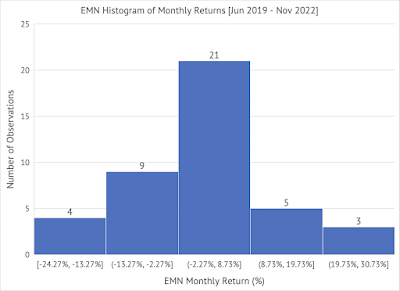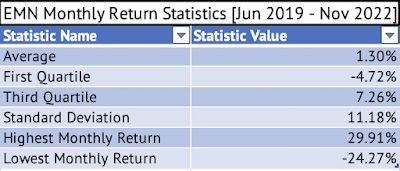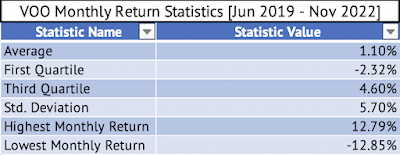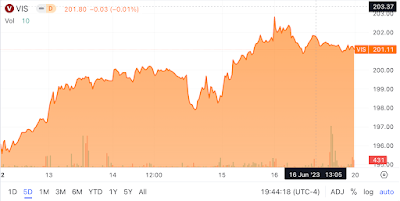## Tuesday, December 13, 2022

### Eastman Chemical's Monthly Returns Have a High Correlation with the Vanguard S&P 500 Index ETF

Here's the histogram of monthly returns for Eastman Chemical (EMN) between June 2019 and November 2022 (Exhibit 1). Please click on the image to see an enlarged version.

Exhibit 1:Eastman Chemical Histogram of Monthly Returns (Source: Data Provided by IEX Cloud, Author Calculations using Microsoft Excel)

The average monthly returns of Eastman Chemical are slightly better than that of the Vanguard S&P 500 Index ETF (Exhibit 2 & 3). But Eastman Chemical has a much higher (nearly double) standard deviation (volatility) of monthly returns than the Vanguard S&P 500 Index ETF.

Exhibit 2:Eastman Chemical Average, First Quartile, Third Quartile, and Standard Deviation of Monthly Returns. (Data Provided by IEX Cloud, Author Calculations Using Microsoft Excel)

Exhibit 3:Vanguard S&P 500 Index ETF Average, First Quartile, Third Quartile, and Standard Deviation of Monthly Returns. (Data Provided by IEX Cloud, Author Calculations Using Microsoft Excel)

Eastman Chemical moves closely with the market since it has a high positive correlation of 0.78.

> cor(VOOandEMN['EMN_Monthly_Return'], VOOandEMN['VOO_Monthly_Return'], method = c("pearson", "kendall", "spearman"))

VOO_Monthly_Return

EMN_Monthly_Return          0.7898654

A linear regression model of the monthly returns of Vanguard S&P 500 Index ETF as the independent variable and Eastman Chemical as the dependent variable yields a beta of 1.54.

> # Conduct the Linear Regression of the Monthly Returns Between \$VOO and \$EMN

> lmVOOEMN = lm(EMN_Monthly_Return~VOO_Monthly_Return, data = VOOandEMN)

> # Present the summary of the results from the linear regression

> summary(lmVOOEMN)

Call:

lm(formula = EMN_Monthly_Return ~ VOO_Monthly_Return, data = VOOandEMN)

Residuals:

Min       1Q   Median       3Q      Max

-0.12433 -0.04969 -0.01148  0.05611  0.13701

Coefficients:

Estimate Std. Error t value  Pr(>|t|)

(Intercept)        -0.003992  0.010914   -0.366  0.716

VOO_Monthly_Return  1.548548  0.190108    8.146  5.02e-10 ***

---

Signif. codes:  0 ‘***’ 0.001 ‘**’ 0.01 ‘*’ 0.05 ‘.’ 0.1 ‘ ’ 1

Residual standard error: 0.06942 on 40 degrees of freedom

Multiple R-squared:  0.6239, Adjusted R-squared:  0.6145

F-statistic: 66.35 on 1 and 40 DF,  p-value: 5.023e-10

The coefficient of VOO_Monthly_Return (slope of the regression line) is the stock's beta. This beta value means that for every 1% change in the monthly returns of the Vanguard S&P 500 Index ETF, Eastman Chemical, on average, changes by 1.54% (monthly). This relationship between the two companies is significant at the 95% confidence interval, given the p-value of 5.02e-10.

This close positive relationship between the two explains why Eastman Chemical has lost 25.6%, while the Vanguard S&P 500 Index ETF (VOO) has lost 14.5%.